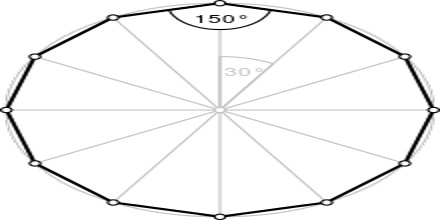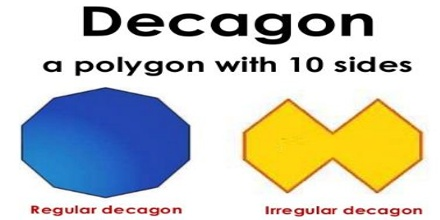Dodecagon: Dodecagon is one of the types of polygon which has twelve sides. There is an important type of polygon which is known as dodecagon. It is a polygon which is bounded by twelve line segments. More specifically, a dodecagon has 12 sides (or edges) and 12 internal (or interior) angles. The sum of the interior angles of a dodecagon is 1800 degrees.

Properties of a Dodecagon

• The total interior angle of a 12 sided polygon is = (12 – 2) 180 degrees = 1800 degrees.
• The total exterior angle of a 12 sided polygon is 360 degrees.
• The internal angle at each vertex of a regular dodecagon is equal to = 1800/12 = 150 degrees. While the exterior angle at a single vertex is; 360/12 = 30 degreesTypes

• Regular Dodecagons
• Irregular Dogecagons

Regular Dodecagons

Regular Dodecagons are those which have all edges equal in length. They have all interior angles also same. Each angle in a regular dodecagon is equal to 150°. So, a dodecagon with all its 12 sides and 12 angles equal is called a regular dodecagon.Irregular Dodecagons

These dodecagons are those 12-sided polygons which do not have all sides equal. An irregular dodecagon does not have all sides and all angles equal.